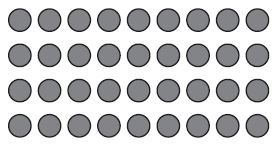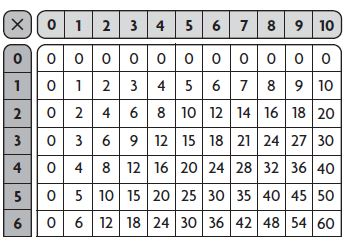# Go Math Grade 3 Answer Key Chapter 4 Multiplication Facts and Strategies Extra Practice

## Go Math Grade 3 Chapter 4 Multiplication Facts and Strategies Extra Practice Answer Key

All concepts are covered in one place along with answers for Go Math Grade 3 Chapter 4 Extra Practice. Follow the steps of Go Math Grade 3 Multiplication Facts and Strategies explanation to learn the easy method to solve problems.

### Common Core – Page No. 87000

Lessons 4.1–4.2

Find the product.

Question 1.
4 × 2 = ______

4 × 2 = 8.

Explanation:
Double 2×2 to get 4×2.
2 x 2 = 4.
Double: 4 + 4 = 8.
4 x 2 = 8.

Question 2.
8 × 5 = ______

8 x 5 = 40

Explanation:
Factor 8 is an even number. 4+ 4
5 x 4 = 20.
20 doubled is 40.
8 x 5 = 40.

Question 3.
10 × 7 = ______

10 × 7 = 70

Explanation:
A multiple of 10 is any product that has 10 as one of its factors. So, the multiplication of any number with 10 is 10’s of that particular number. The answer is 10 × 7 = 70.

Question 4.
2 × 9 = ______

2 x 9 = 18

Explanation:
Double the given number 6 to get the final answer. The answer is 9 + 9 = 18.
2 x 9 = 18.

Question 5.
6
× 1 0
——-
______

6 x 10 = 60

Explanation:
Using doubles, we can find a 6 x 10 value. First, multiply the factor with half of 6. So, now we can do 3 x 10 = 30. Now, we can double the value of 3 x 10. That is 30 + 30 = 60. So, the answer for 6 x 10 = 60.

Question 6.
5
× 7
——-
______

5 x 7 = 35.

Explanation:

Skip count by 5’s until you say 7 numbers. 5, 10, 15, 20, 25, 30, 35. Now, the count of the number is 7. So, the answer for 5 x 7 is 35.

Question 7.
2
× 1 0
——-
______

2 x 10 = 20.

Explanation:
Double 10 to get the answer of 2 x 10.
10 + 10 = 20.
2 x 10 = 20.

Question 8.
4
× 5
——-
______

4 x 5 =20

Explanation:
Multiply 2×5 to get the answer for 4×5. Double the answer of 2×5 to get the final answer.
2 x 5 =10.
Double: 10 + 10 = 20.
4 x 5 =20.

Lessons 4.3–4.5

Find the product.

Question 9.
6
× 2
——-
______

6 x 2 = 12.

Explanation:
Use doubles to find the answer of 6 x 2.
Multiply 3 x 2 = 6.
Double: 6 + 6 = 12.
The answer for 6 x 2 is 12.

Question 10.
3
× 9
——-
______

3 x 9 = 27.

Explanation:

Skip count by 3’s until you say 9 numbers. Write like 3, 6, 9, 12, 15, 18, 21, 24, 27. The answer for 3 x 9 is 27.

Question 11.
7
× 3
——-
______

7 x 3 = 21

Explanation:
Write 7 x 3 as 3 x 7 according to the Commutative Law of Multiplication.
Skip count by 3’s until you say 7 numbers. Write like 3, 6, 9, 12, 15, 18, 21. The answer for 3 x 7 is 21. So, 7 x 3 = 21.

Question 12.
8
× 6
——-
______

8 × 6 = 48

Explanation:
8 × 6 = (2 x 4) x 6
Use the Associative Property.
8 × 6 = 2 x (4 x 6)
Multiply. 4 × 6
8 × 6 = 2 x 24
Double the product.
8 × 6 = 24 + 24
8 × 6 = 48.

Write one way to break apart the array. Then find the product.

Question 13.Type below:
__________

36

Explanation:
Draw a line to seperate the columns. Divide the columns with 4 and 5 factors.
The array is 4 x 9 = 4 x (5 + 4)
(4 x 5) + (4 x 4) = 20 + 16 = 36.

Find the product.

Question 14.
5 × 7 = ______

5 x 7 = 35

Explanation:
Skip count by 5’s until you say 7 numbers. 5, 10, 15, 20, 25, 30, 35. Now, the count of the number is 7. So, the answer for 5 x 7 is 35.

Question 15.
2 × 6 = ______

2 x 6 = 12

Explanation:
Double 6 to get the answer. 6 + 6 = 12.
2 x 6 = 12.

Question 16.
4 × 7 = ______

Double 2 x 7 to find 4 x 7.
2 x 7 = 14.
Double: 14 + 14 = 28.
4 x 7 = 28.

Explanation:

Question 17.
8 × 3 = ______

8 × 3 = 24

Explanation:
8 × 3 = (2 x 4) x 3
Use the Associative Property.
8 × 3 = 2 x (4 x 3)
Multiply. 4 × 3
8 × 3 = 2 x 12
Double the product.
8 × 3 = 12 + 12
8 × 3 = 24.

Question 18.
Abby has 5 stacks of cards with 7 cards in each stack. How many cards does she have in all?
______ cards

35 cards

Explanation:
5 x 7 = 35.
Abby has 35 cards.

Question 18.
Noah has 3 sisters. He gave 6 balloons to each sister. How many balloons did Noah give away in all?
______ balloons

18 balloons

Explanation:
3 x 6 = 18.
Noah gave 18 balloons to his sisters.

### Common Core – Page No. 88000

Lesson 4.6

Write another way to group the factors. Then find the product.

Question 1.
(3 × 2) × 5 = ______
Explain:
__________

3 × (2 × 5)
30

Explanation:
Using Associative Property of Multiplication, we can write (3 × 2) × 5 = 3 × (2 × 5).
Find (3 × 2) × 5. Multiply 3 x 2 = 6. Then, multiply 6 x 5 = 30.
Find 3 x (2 x 5). Multiply 2 x 5 = 10. Then, multiply 3 x 10 = 30.
So, (3 × 2) × 5 = 3 × (2 × 5). The product value is 30.

Question 2.
2 × (5 × 3) = ______
Explain:
__________

(2 x 5) x 3
30

Explanation:
Using the Associative Property of Multiplication, we can write 2 × (5 × 3) = (2 x 5) x 3.
Find 2 × (5 × 3). Multiply 5 x 3 = 15. Then, multiply 2 x 15 = 30.
Find (2 x 5) x 3. Multiply 2 x 5 = 10. Then, multiply 10 x 3 = 30.
So, 2 × (5 × 3) = (2 x 5) x 3. The product value is 30.

Question 3.
(1 × 4) × 2 = ______
Explain:
__________

1 x (4 x 2)

Explanation:
Using the Associative Property of Multiplication, we can write (1 × 4) × 2 = 1 x (4 x 2).
Find 1 × (4 × 2). Multiply 4 x 2 = 8. Then, multiply 1 x 8 = 8.
Find (1 x 4) x 2. Multiply 1 x 4 = 4. Then, multiply 4 x 2 = 8.
So, (1 × 4) × 2 = 1 x (4 x 2). The product value is 8.

Lesson 4.7

Is the product even or odd?
Write even or odd.Question 4.
6 × 6
______

Even

Explanation:
6 x 6 = 36. The numbers end with 0, 2, 4, 6, 8 are even numbers. So, 36 is even number. The 6 x 6 is an even number.

Question 5.
2 × 3
______

even

Explanation:
Products with 2 as a factor are even.

Question 6.
3 × 9
______

odd

Explanation:
The product of two odd numbers is an odd number. The answer is odd. So, 3 x 9 = 27.

Lessons 4.8–4.9

Find the product.

Question 7.
8 × 2 = ______

16

Explanation:
Factor 8 is an even number. 4+ 4
2 x 4 = 8.
8 doubled is 16.
8 x 2 = 16.

Question 8.
5 × 9 = ______

5 × 9 = 45

Explanation:
The multiplication of 5 × 9 is calculated as Skip-count by 5’s 9 times. You can write as 5, 10, 15, 20, 25, 30, 35, 40, 45. The final answer for 5 × 9 is 45.

Question 9.
______ = 3 × 9

27 = 3 x 9

Explanation:
Skip count by 3’s until you say 9 numbers. Write like 3, 6, 9, 12, 15, 18, 21, 24, 27. The answer for 3 x 9 is 27.

Question 10.
4 × 8 = ______

2 × 5 = 10

Explanation:
Double 2×8 to get 4×8.
2 x 8 = 16.
16 + 16 = 32.
4 x 8 = 32.

Question 11.
______ = 9 × 4

36

Explanation:
9 = 3 + 6
9 × 4 = (3 + 6) x 4
9 × 4 = (3 x 4) + (6 x 4)
9 × 4 = 12 + 24
9 × 4 = 36.

Question 12.
6 × 8 = ______

48

Explanation:
Use doubles to find the answer of 6 x 8. Firstly, multiply 3 x 8 = 24. Then, double the value of 3 x 8. 24 + 24 = 48. The answer for 6 x 8 is 48.

Lesson 4.10

Question 13.
Leo has a total of 45¢. He has some dimes and pennies. How many different combinations of dimes and pennies could Leo have? Make a table to solve.
Leo could have ______ combinations of 45¢.

Leo could have 4 combinations of 45¢.

Explanation:
1 dime 35 pennies
2 dimes 25 pennies
3 dimes 15 pennies
4 dimes 5 pennies.

Question 13.

 Number of Dimes ____1_____ _____2____ ___3______ _____4____ Number of Pennies ____35_____ _____25____ ___15______ ______5___ Total Value 45¢ 45¢ 45¢ 45¢

### Conclusion

Here is the comprehensive collection of math solutions for Grade 3 Chapter 4 Multiplication Facts and Strategies. Go Math Grade 3 Answer Key Chapter 4 Multiplication Facts and Strategies Extra Practice is the source for students to learn easy maths. Follow the methods of solving problems mentioned on this page and get the Go Math Grade 3 Chapter 4 Multiplication Facts and Strategies Extra Practice Answer Key.

Scroll to Top